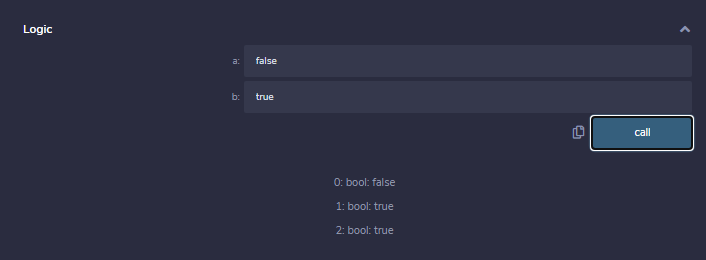# Solidity – Operators

• Difficulty Level : Easy
• Last Updated : 11 May, 2022

In any programming language, operators play a vital role i.e. they create a foundation for the programming. Similarly, the functionality of Solidity is also incomplete without the use of operators. Operators allow users to perform different operations on operands. Solidity supports the following types of operators based upon their functionality.

1. Arithmetic Operators
2. Relational Operators
3. Logical Operators
4. Bitwise Operators
5. Assignment operators
6. Conditional Operator

### Arithmetic Operators

These operators are used to perform arithmetic or mathematical operations. Solidity supports the following arithmetic operators :

Example: In the below example, the contract SolidityTest demonstrates the above mentioned different types of arithmetic operators.

## Solidity

 `// Solidity contract to demonstrate``// Arithmetic Operator``pragma solidity ^0.5.0;` `// Creating a contract``contract` `SolidityTest {` `    ``// Initializing variables``    ``uint16 ``public` `a = 20;``    ``uint16 ``public` `b = 10;` `    ``// Initializing a variable``    ``// with sum``    ``uint ``public` `sum = a + b;` `    ``// Initializing a variable``    ``// with the difference``    ``uint ``public` `diff = a - b;` `    ``// Initializing a variable``    ``// with product``    ``uint ``public` `mul = a * b;` `    ``// Initializing a variable``    ``// with quotient``    ``uint ``public` `div` `= a / b;` `    ``// Initializing a variable``    ``// with modulus``    ``uint ``public` `mod = a % b;` `    ``// Initializing a variable``    ``// decrement value``    ``uint ``public` `dec = --b;` `    ``// Initializing a variable``    ``// with increment value``    ``uint ``public` `inc = ++a;``    ` `}`

Output :### Relational Operators

These operators are used to compare two values. Solidity supports the following relational operators :

Example: In the below example, the contract SolidityTest demonstrates the above mentioned different types of relational operators.

## Solidity

 `// Solidity program to demonstrate``// Relational Operator``pragma solidity ^0.5.0;` `// Creating a contract``contract` `SolidityTest {` `    ``// Declaring variables``    ``uint16 ``public` `a = 20;``    ``uint16 ``public` `b = 10;` `    ``// Initializing a variable``    ``// with bool equal result``    ``bool` `public` `eq = a == b;` `    ``// Initializing a variable``    ``// with bool not equal result``    ``bool` `public` `noteq = a != b;``   ` `    ``// Initializing a variable``    ``// with bool greater than result``    ``bool` `public` `gtr = a > b;` `    ``// Initializing a variable``    ``// with bool less than result``    ``bool` `public` `les = a < b;` `    ``// Initializing a variable``    ``// with bool greater than equal to result``    ``bool` `public` `gtreq = a >= b;` `    ``// Initializing a variable``    ``// bool less than equal to result``    ``bool` `public` `leseq = a <= b;``    ` `}`

Output :### Logical Operators

These operators are used to combine two or more conditions. Solidity supports the following arithmetic operators :

Example: In the below example, the contract logicalOperator demonstrates the above mentioned different types of logical operators.

## Solidity

 `// Solidity program to demonstrate``// Logical Operators``pragma solidity ^0.5.0;` `// Creating a contract``contract` `logicalOperator{` `     ``// Defining function to demonstrate``     ``// Logical operator``     ``function Logic(``       ``bool` `a, ``bool` `b) ``public` `view returns(``       ``bool``, ``bool``, ``bool``){``       ` `       ``// Logical AND operator ``       ``bool` `and = a&&b;``       ` `       ``// Logical OR operator ``       ``bool` `or = a||b;``       ` `       ``// Logical NOT operator``       ``bool` `not = !a;``       ``return` `(and, or, not);`` ``}``}`

Output :### Bitwise Operators

These operators work at a bit level used to perform bit-level operations. Solidity supports the following arithmetic operators :

Example: In the below example, the contract SolidityTest demonstrates the above mentioned different types of bitwise operators.

## Solidity

 `// Solidity program to demonstrate``// Bitwise Operator``pragma solidity ^0.5.0;` `// Creating a contract``contract` `SolidityTest {` `    ``// Declaring variables``    ``uint16 ``public` `a = 20;``    ``uint16 ``public` `b = 10;` `    ``// Initializing a variable``    ``// to '&' value``    ``uint16 ``public` `and = a & b;` `    ``// Initializing a variable``    ``// to '|' value``    ``uint16 ``public` `or = a | b;` `    ``// Initializing a variable``    ``// to '^' value``    ``uint16 ``public` `xor = a ^ b;` `    ``// Initializing a variable``    ``// to '<<' value``    ``uint16 ``public` `leftshift = a << b;` `    ``// Initializing a variable``    ``// to '>>' value``    ``uint16 ``public` `rightshift = a >> b;``  ` `    ``// Initializing a variable``    ``// to '~' value``    ``uint16 ``public` `not = ~a ;``    ` `}`

Output :### Assignment Operator

These operators are for the assignment of value to a variable. The operand at the left side is variable while operand at the right side is value. Solidity supports the following arithmetic operators :

Example: In the below example, the contract SolidityTest demonstrates the above mentioned different types of assignment operators.

## Solidity

 `// Solidity program to demonstrate``// Assignment Operator``pragma solidity ^0.5.0;` `// Creating a contract``contract` `SolidityTest {` `        ``// Declaring variables``        ``uint16 ``public` `assignment = 20;``        ``uint ``public` `assignment_add = 50;``        ``uint ``public` `assign_sub = 50;``        ``uint ``public` `assign_mul = 10;``        ``uint ``public` `assign_div = 50;``        ``uint ``public` `assign_mod = 32;``    ` `        ``// Defining function to``        ``// demonstrate Assignment Operator``        ``function getResult() ``public``{``           ``assignment_add += 10;``           ``assign_sub -= 20;``           ``assign_mul *= 10;``           ``assign_div /= 10;``           ``assign_mod %= 20;``           ``return` `;``        ``}``}`

Output :### Conditional Operators

It is a ternary operator that evaluates the expression first then checks the condition for return values corresponding to true or false.

Syntax:

`if condition true ? then A: else B`

Example: In the below example, the contract SolidityTest demonstrates the conditional operator.

## Solidity

 `// Solidity program to demonstrate``// Conditional Operator``pragma solidity ^0.5.0;` `// Creating a contract``contract` `SolidityTest{` `     ``// Defining function to demonstrate``     ``// conditional operator``     ``function sub(``       ``uint a, uint b) ``public` `view returns(``       ``uint){``      ``uint result = (a > b? a-b : b-a);``      ``return` `result;`` ``}``}`

Output :My Personal Notes arrow_drop_up